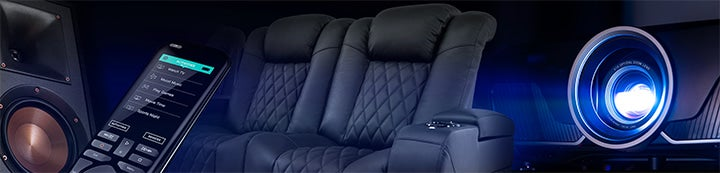1 - 2 of 2 Posts

#### DCJ98GST

·
##### Registered
Joined
·
143 Posts
Discussion Starter · ·
I own two Parasound HCA-2200 amplifiers. I currently run one in stereo mode. These amps bias the first 6 watts in pure class A amplification per channel (12 watts total).

If I bridge them to run one 2200 each channel, how much class A amplification would I get each channel? I am using 8ohm speakers.

1. 12 watts class A each channel (24 watts total) ?

2. 6 watts class A each channel (12 watts total) ?

I do not really understand how bridging would affect this, if somebody could explain the concept, please let me know.

Thanks!

#### MichaelJHuman

·
##### Registered
Joined
·
18,923 Posts
Well, it's pretty simple, I think?

Bridging doubles the potential across each output terminal. So say the max unclipped voltage at the output terminal unbridged, while still in class A was 6 watts. The formula for power in this case is v*v/r. Assuming 8 ohms, voltage is 6.9 Volts.

Now when you bridge two channels, you double that, as you are increasing potential by inverting one channel (so if one channel was +6.9 Volts, the other would be -6.9 Volts.) 13.8 Volts total potential.

Cranking that back into v*v/r, you get 23.8 Watts. This is as expected, as power should go up by 4 when bridged as memory serves (and this math should show why.)

1 - 2 of 2 Posts